## Estimation of Taylor Series

Suppose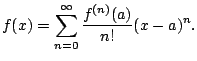Write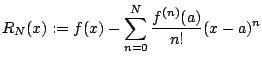We call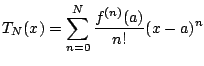the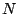th degree Taylor polynomial. Notice that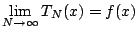if and only if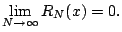We would like to estimate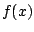with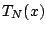. We need an estimate for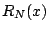.

Theorem 6.7.1 (Taylor's theorem)   If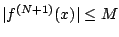for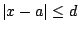, then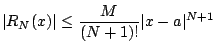for $|x-a|&le#leq;d$.

For example, if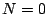, this says that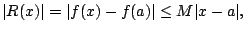i.e.,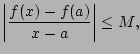which should look familiar from a previous class (Mean Value Theorem).

Applications:

1. One can use Theoremto prove that functions converge to their Taylor series.

2. Returning to the relativity example above, we apply Taylor's theorem with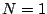and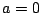. With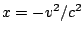and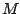any number such that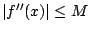, we have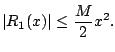For example, if we assume that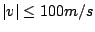we use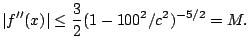Using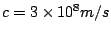, we get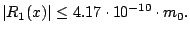Thus for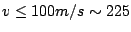mph, then the error in throwing away relativistic factors is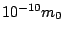. This is like 200 feet out of the distance to the sun (93 million miles). So relativistic and Newtonian kinetic energies are almost the same for reasonable speeds.

William Stein 2006-03-15